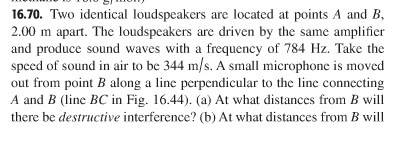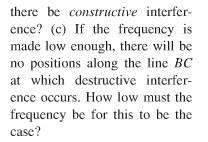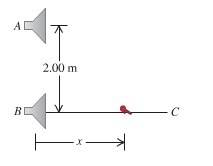# Destructive Interference

rteng

## Homework Statement## Homework Equations

destructive interference occurs at 0.5(lambda), (3/2)(lambda),...

## The Attempt at a Solution

I found the path difference to be:
d=sqrt(4+x^2)-x

and this has to be equal to (n)(lambda)/2 where n is an odd integer for destructive interference

this does not work out algebraically for me...as the x^2-x^2=0

is this path difference not correct?

do I just use f(n)=(nv)/(2d) to find d?

Why did you do: x^2-x^2=0? Where does that come from?

rteng
ok I found my path difference to be sqrt(4+x^2)-x

so if I equate this to (n)(lambda)/2

then: sqrt(4+x^2)-x=(n)(lambda)/2

4+x^2-x^2=(n^2)(lambda^2)/4

there is my problem...

Do you know how to find lambda?

Oh, (sqrt(4 + x^2) - x)^2 = (sqrt(4 + x^2) - x) * (sqrt(4 + x^2) - x)
You don't just square the terms.

rteng
ah yes...of course

I think that should be the proper way to solve this problem...at least for a and b

now on to c...

rteng
ok I do not understand c
can anybody help?

rteng
I would say that the only way for which there be no destructive interference is if x is 0
but that revelation is really vague for me...could it be correct?

rteng
the I get f=86Hz